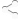# Help with a math problem4 replies to this topic

### #1Jake Posted August 02, 2012 - 09:15 PM

I literally have one problem left on my summer homework. I worked everything else out, but I can't figure this out no matter how hard I try. Could someone tell me the steps needed to solve it?Solve for x: 2/3x-4=1/2(3x-5)Elder Souls
The discussion continues after this sponsored message. Tired of seeing advertisements? Create an account to remove them.

### #2David Posted August 02, 2012 - 09:58 PM

x = -1.8First multiply the 1/2 on the right by both values inside the parentheses to get rid of them.(2/3)x - 4 = 1.5x - 2.5Then subtract (2/3)x from (1.5)x to get one x value. (9/6) - (4/6) = (5/6)-4 = (5/6)x - 2.5Add 2.5 to each side to get the x alone.-1.5 = (5/6)xDivide each side by (5/6).-1.8 = xCheck by plugging -1.8 in for x in the original equation.(2/3)(-1.8) - 4 = -5.2(1/2)( 3(-1.8) - 5 ) = -5.2Success.-5.2 = -5.2

### #3Jake Posted August 02, 2012 - 11:58 PM

Thanks, Traag. I tried to do the problem while I waited for a response, and my original answer was completely wrong.EDIT: By the way, I'm almost finished with my new article. I just need to figure out how to insert the pictures...

### #4Carroll 1 Posted August 03, 2012 - 12:07 AMThis is an alternative method, With the same answer so i believe it is correct. This is the way I was taught in college to solve mathmatical equations (using fractions.) You may find this easier for you, hence me posting my work.]

Step 1 Multiply "1/2" into the bracket containing (3x-5) using the numerator and keeping the original denominator along with carrying over the "X" with your first value. Using BEDMAS of course (Brackets, Exponents, Multiplication/Divison, Adding/Subtraction)
Step 2 Rewrite your equation and check your work ensuring the process was properly completed.
Step 3 Determin your LCM (Least Common Multiple) which can usually be done by multiplying your denominators in the equation (in this case the LCM is 6, 2x3). After finding your LCM multiply each value by this number and any value that has a Denominator other then "1" (any whole number with no visable denominator is considered to be "over" 1) is first multiplied by the LCM then divided by the demoninator.
Step 4 Rewrite your equation and check your work ensuring the process was properly completed.
Step 5 Rearrange this equation grouping the values with the "Constant" ( I think is the word i'm looking for) "X" on one side of the Equals sign (remembering to change the negative or positive if it crosses sides of the equals sign) and the whole numbers grouped on the other following the same principal.
Step 6 Carry out the math involved in Step 5 and divide the number that is included beside the Constant "-5" in this case, to itself and the whole number provided after carrying out the simple math on the right side of the equals sign. (Remember to bring the pos/neg sign across as well)
Step 7 Rewrite your equation in it's simplest form and check your divison in Step 6.

The way Traagien did it is just as right as mine. For me I find this easier. I hope my explaination provided the help you needed to find your mistakes. I explained everything in some sort of "detail" for the purpose as to aid your learning curve. Not patornize your intelligence.

For myself I found steps two, four, and six helped me correct silly mistakes. Helped greatly on my Block 1 Exam for Electrical.

### #5Huygens Posted August 03, 2012 - 03:03 AM

Damn, summer homework, I've literally never ever heard of that. I hope it doesn't trouble you too much, enjoy the summer while you still can!

#### 0 user(s) are reading this topic

0 members, 0 guests, 0 anonymous users